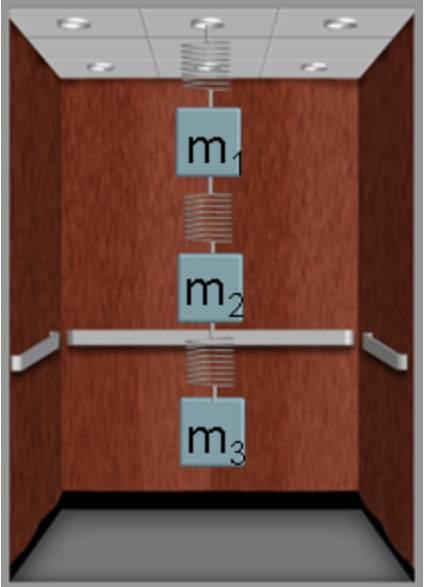# Spring Problem with 3 springs suspending 3 masses in series in an elevator

• Hippo89
Hippo89
Homework Statement
What is the distance the upper spring is streched?
Relevant Equations
Fnet = mam1 = 4 kg, m2 = 12 kg, m3 = 8 kg. k = 327 N/m for all three blocks. The elevator accelerates upwards at 3.8 m/s^2.

Net force of block one would be equal to force applied by top spring minus weight of system, since top spring is holding all 3 blocks.
F1 = 4*3.8= Fs,top - Wsystem = Fs,top - 24*9.8. Fs,top = 250.4 N = -327x. x = -.76 m displacement

The correct answer is -1 m.

Homework Helper
Gold Member
The force acting downward on block m1 is not the weight of all three blocks. The weight of block m1 acts downward on block m1. There is one other force that acts downward on block m1. Can you identify this other force?

Homework Helper
Gold Member
Here is another way to look at it. We are assuming that the three masses accelerate as one, i.e. they have the same acceleration at all times.

What if you took the three blocks together as your system?
What is the net force on the three-mass system?
What forces acting on the three-mass system are external to it and contribute to the net force?
What does Newton's second law say about all this?

Hippo89
Here is another way to look at it. We are assuming that the three masses accelerate as one, i.e. they have the same acceleration at all times.

What if you took the three blocks together as your system?
What is the net force on the three-mass system?
What forces acting on the three-mass system are external to it and contribute to the net force?
What does Newton's second law say about all this?
Okay. I treated the system as all 3 blocks accelerating together at 3.8 m/s^2, since the top spring is carrying all three blocks.
Fnet,system = (m, system)(a) = Force of upper spring - Weight of all three blocks.
With these calculations I got a downwards displacement of 1 meter, which is the correct answer.

There was another question in this set of problems. Under these conditions, what is the distance the middle spring is streched?

I used the same logic that helped me answer the original question. I defined the blocks the spring was carrying as the system, which for the middle spring, would be blocks 2 and 3.
Fnet, system = (m2+m3)(a) = Force of middle spring - Weight of blocks 2 and 3.
With these calculations, I got a downwards displacement of 0.83 m, which apparently is the wrong answer.

What am I missing for this other question?

Gold Member
I used the same logic that helped me answer the original question. I defined the blocks the spring was carrying as the system, which for the middle spring, would be blocks 2 and 3.
Fnet, system = (m2+m3)(a) = Force of middle spring - Weight of blocks 2 and 3.
With these calculations, I got a downwards displacement of 0.83 m, which apparently is the wrong answer.

What am I missing for this other question?
What is apparently the correct answer?

Homework Helper
Gold Member
I get the same answer as you to two sig figs.

•erobz
Hippo89
What is apparently the correct answer?
The website I’m using, Flipit, only states if you got the right or wrong answer. So I don’t know the correct answer.

Gold Member
The website I’m using, Flipit, only states if you got the right or wrong answer. So I don’t know the correct answer.
I think, "we" ( @kuruman and I ) think the website is incorrect.

Homework Helper
Gold Member